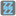• # NameError: name 'input' is not defined

## Question related to missionFizz Buzz

I keep getting the NameError when trying to run this code, what am I missing?

```#Your optional code here
#You can import some modules or create additional functions

def checkio(number):
if number < 0:
return 'no negative numbers'
elif number % 3 == 0 and num % 5 == 0:
return 'Fizz Buzz'
elif number % 3 == 0:
return 'Fizz'
elif number % 5 == 0:
return 'Buzz'
else:
return str(number)

num = int(input('Enter number:'))

checkio(num)

#Some hints:
#Convert a number in the string with str(n)

#These "asserts" using only for self-checking and not necessary for auto-testing
if __name__ == '__main__':
assert checkio(15) == "Fizz Buzz", "15 is divisible by 3 and 5"
assert checkio(6) == "Fizz", "6 is divisible by 3"
assert checkio(5) == "Buzz", "5 is divisible by 5"
assert checkio(7) == "7", "7 is not divisible by 3 or 5"
```5